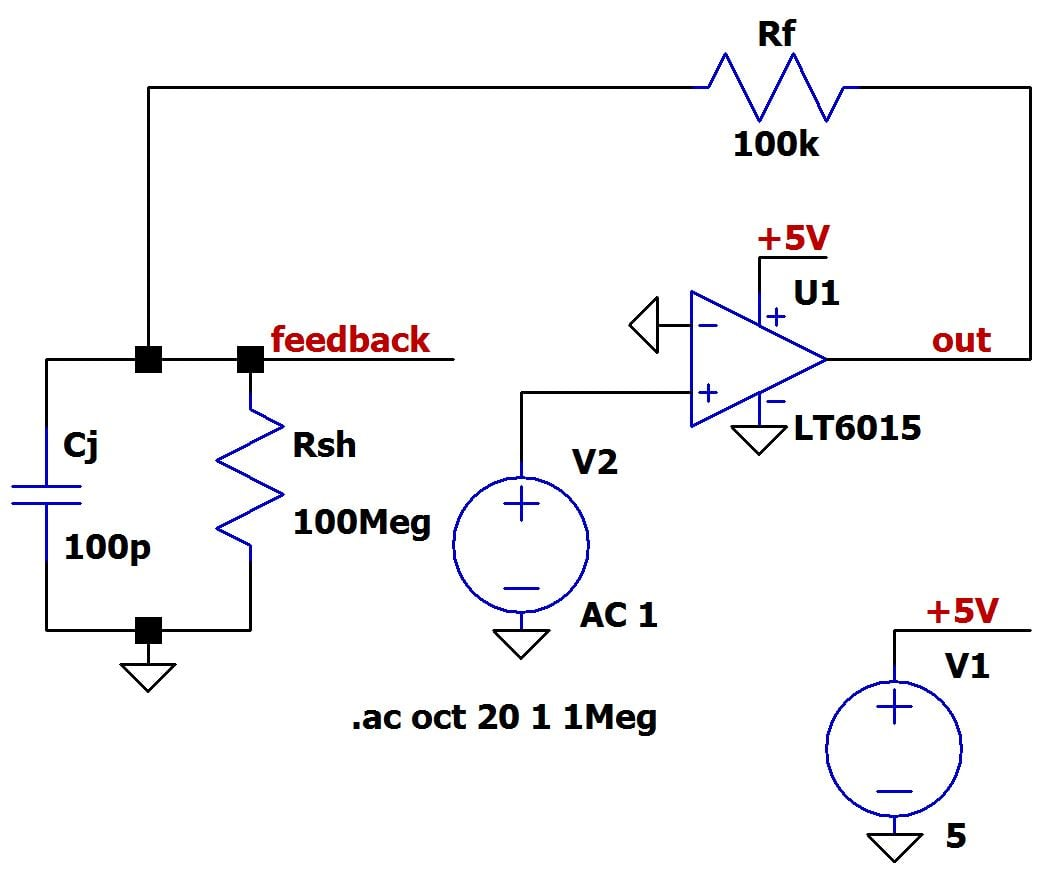# Open-loop gain of Op-amp - LT6015

I am simulating the LTSpice circuit, as seen below, of the transimpedance amplifier using the LT6015 Op-Amp as depicted in the article.However when I run the simulation I am not able to get the same Open-loop frequency response as stated in the article.

I obtain a max open-loop gain of ~145dB, whereas he has ~102dB. Furthermore I created some matlab code to check the open-loop gain using the approximation of the op-amp as a first-order low-pass filter. Taken from the datasheet of the LT6015 the open-loop gain is 3,000,000 and the open-loop bandwidth is therefore 1.1Hz (calculated from the gain bandwidth product, with a value of 3.2MHz). However this outputs a open-loop gain of ~129dB.

Here is the code of my matlab program:

    Ao = 3000*10^3 %Open-loop gain
Wb = 1.1 %Open-loop bandwidth of op-amp

w = .1:.1:100000;
h = 20*log10(Ao*(abs(1./(1+(j*w)/(Wb)))))

figure
semilogx(w,h) %% Plot on log scale
hold on
ylabel('|H(j\omega)|dB')
legend('1','2')


I am unable to see what I am doing wrong to create the discrepancy between these three plots, with the primary focus being the former two, the last one acts as check. Perhaps the last one is incorrect because the LT6015 cannot be modelled as first-order low-pass filter?

Any help would be greatly appreciated!

• I doubt if you can simulate open loop gain without negative DC feedback (or without offset correction). More than that, why do you use single supply only? Which operational point do you expect?
– LvW
Jun 20, 2016 at 13:01
• I agree with LvW about the DC feedback, the article's way of doing things will only work with a (sort of) ideal model of the opamp, it would not with a more realistic (transistor level) model. Also the article also does not provide enough detail to guide a beginner to doing this properly. Are you sure you're using the same model as in the article ? In practice open loop gain also does not matter much as we use opamps in feedback mode mainly. Jun 20, 2016 at 13:08
• @LvW - But surely open loop gain, as in the name, is not a closed loop, and therefore the feedback included? The idea is to convert the current signal to voltage, which should be positive and therefore the negative voltage supply is not required? FakeMoustache Both are the LT6015 available from the LTSpice 'component' key. But in stability analysis it is important, as it allows you to compare it's slope with the slope of the 1/B (feedback term) and therefore determine if it is stable? Jun 20, 2016 at 13:29
• ConfusedCheese, this is a common problem: For open-loop measurements (or real world simulations) we need some DC feedback. That means: A frequency-selective feedback path with a very low cutoff frequency (some Hz only).See my answer (and a circuit) here: electronics.stackexchange.com/questions/241729/…
– LvW
Jun 20, 2016 at 14:14

ConfusedCheese, here is a simple method for simulating the loop gain for an operational amplifier with feedback. This simulation scheme provides DC feedback and a stable operational point (for double and symmetrical voltage supply only!).

• The dashed box contains the feedback factor, if any!.

• Without this box (direct connection to the inv. input) you simulate the open-loop gain Aol of the opamp only.

• The loop gain is simply T(s)=V(node2)/V(node1). Of course, the frequency must be swept over the desired range (ac analysis).simulate this circuit – Schematic created using CircuitLab

• I am very confused about where to keep what and where to apply the voltage source, multiple different sources are telling me different things. Above it is saying to keep it all the same but insert a voltage source between the output and feedback network, but a post above is saying to implement a much more complicated method. Jun 20, 2016 at 15:04
• I am looking to plot the open-loop gain and the inverse of the feedback factor to allow me to analyse the stability of the transimpedance amplifier by looking at the difference in the slopes in their frequency response at their intersect? Jun 20, 2016 at 15:05
• For simulation, the method as shown in the figure above can be used. For real measurtements, this NOT possible and a somewhat more complicated feedback arrangement must be used. For stability analyses it is NOT necessary to plot the opamps gain and the inverse feedback factor separately. You can, from the beginning, plot the loop gain of the whole loop (under open conditions) because the stability criterion applies to this gain of the open loop only.
– LvW
Jun 20, 2016 at 15:47
• @ConfusedCheese The method described by LvW is a semplified version of a well-known method to find the loop-gain developed by Middlebrook, and described in this paper. The semplification works when you connect the voltage source (V1 in the schematic above) to a low-impedance node, like the output of an op amp. In the more general case, the method requires two injections, a voltage injection and a current one. Jun 20, 2016 at 18:36
• Yes - Massimo Ortolano, you are right. It is a special version of the Middlebrook method. In this case (low impedance node at the opamp outpt) a current-injection is not necessary. But again: It works for simulation only (because of the floating voltage source).
– LvW
Jun 20, 2016 at 18:55